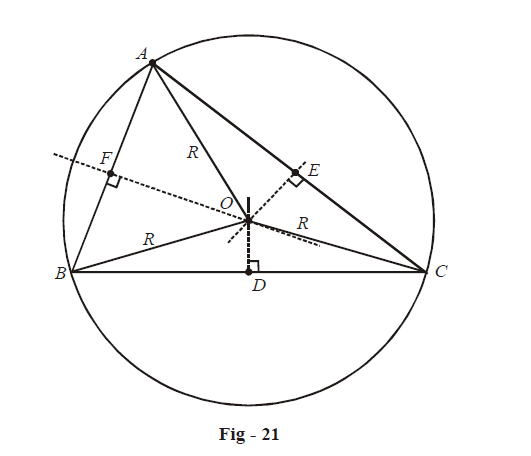# Circumcircle Formulae

### Property - 4: Circumcircle, Incircle, Excircle relations

The radius of the circumcircle of a triangle  $$\Delta ABC$$  is generally denoted as R. Recall how we can construct the circumcircle, by first determining its center as the point of concurrency of the perpendicular bisectors of the sides of the triangle.The next two relations are concerned with R :

\begin{align} \boxed {\;R = \frac{a}{{2\sin A}} = \frac{b}{{2\sin B}} = \frac{c}{{2\sin C}}\; \\ \;R = \frac{{abc}}{{4\Delta }}} \end{align}\!\!\begin{gathered}\text {Important !}\end{gathered}

Proofs: Note that  \begin{align}\angle BOD = \frac{1}{2}\angle BOC = \frac{1}{2}(2\angle A) = \angle A\end{align}

Applying the sine rule in  $$\Delta BOD$$ , we have

\begin{align}&\frac{R}{{\sin \angle BDO}} = \frac{{BD}}{{\sin \angle BOD}} \\ \Rightarrow\quad &\frac{R}{{\sin \begin{align}\left( {\frac{\pi }{2}} \right)\end{align}}} = \frac{{a/2}}{{\sin A}} \\ \Rightarrow\quad & R = \frac{a}{{2\sin A}} \\ & R = \frac{b}{{2\sin B}} = \frac{c}{{2\sin C}}\\ \end{align}

Similarly,

Incidentally, the sine rule can now be re-written as

$\frac{a}{{\sin A}} = \frac{b}{{\sin B}} = \frac{c}{{\sin C}} = 2R$

To prove the second relation, we have

\begin{align}&\,\,\,\,\,\,\,\,\,\,\,\,\,\,\,\,\,\,\,\Delta = \frac{1}{2}bc\,\sin A \Rightarrow \sin A = \frac{{2\Delta }}{{bc}} \\ &\Rightarrow\qquad R = \frac{a}{{2\sin A}} = \frac{a}{{2\left( {\frac{{2\Delta }}{{bc}}} \right)}} = \frac{{abc}}{{4\Delta }} \\ \end{align}